# Generate Random numbers is c#

Wikitechy | 1825 Views | c# | 06 Jun 2016

### Random class :

• All possible Pseudo-random numbers with equal probability from a set of finite numbers can be achieved in c# using random class.
• A clear mathematical computation is done with the help of Knuth subtractive random number generator algorithm.
Here is the small piece of code to generate random numbers in c#

```using System;
using System.Collections.Generic;
using System.Linq;
using System.Text;
namespace random_number
{
class Program
{
static void Main(string[] args)
{
Random rnd = new Random();
for (int i = 0; i < 10; i++)
{  // print random numbers
Console.WriteLine(GenerateDigit(rnd));
}
}
static int GenerateDigit(Random rnd)
{// static method to generate random numbers and return integer
// values using random.next method.
return rnd.Next(500);
}
}
}
```
Code Explanation :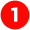Indicates the system namespace.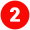Indicates the custom user defined namespace. It can be changed and it’s created once we create the c# project.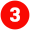Class name of the program which we’ve created. In our class, the class name is Program.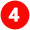All programs will start executing from a standard starting point that’s static void Main(string[] args) function.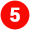Random function is used to generate random numbers. Rnd is the object created for generating random numbers. Random rnd = new Random();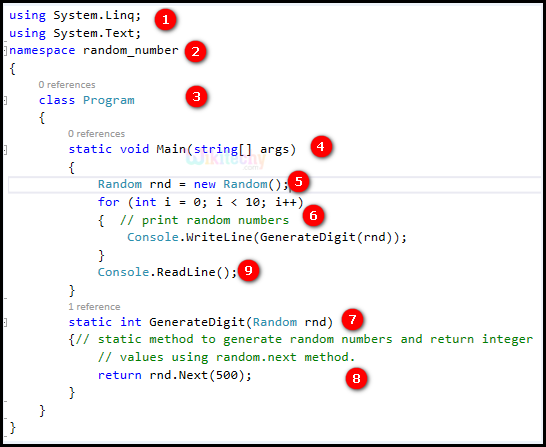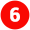A for loop to 10 times to print the random numbers generated by the method GenerateDigit(Random rnd)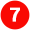Function call  GenerateDigit method is called static int GenerateDigit(Random rnd)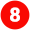Rnd.Next(500) will try to generate random number from 0 to 500 and its returned as function return value.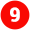This makes us to hold the output window to see the output. Waiting for the user input is the exact meaning of the statement.

Output:

10 random numbers between 0 to 500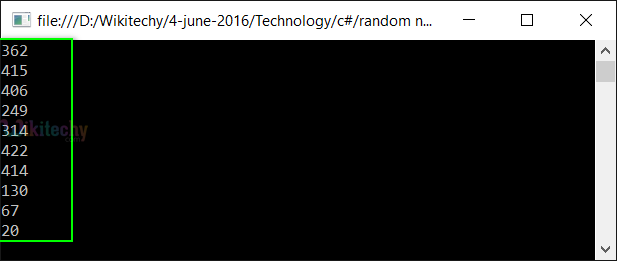Bug Bounty
IOT Hackathon
Webinar